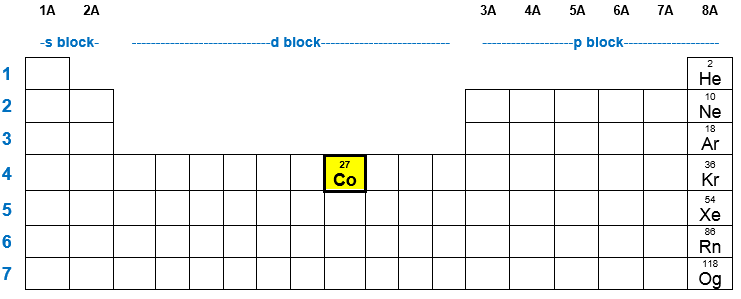# Problem: a. How many electrons does a Co atom have in its 3d subshell?b. How many of those electrons are unpaired?

84% (34 ratings)
###### FREE Expert Solution

We are being asked how many electrons are present in the 3d subshell of Co atom and how many of those electrons are unpaired. Let’s first write the electron configuration of Co atom.

Neutral element:       Atomic number = # protons = # of electronsCobalt (Co):

Atomic number = 27
# of electrons = 27 e-

84% (34 ratings)###### Problem Details

a. How many electrons does a Co atom have in its 3d subshell?

b. How many of those electrons are unpaired?

What scientific concept do you need to know in order to solve this problem?

Our tutors have indicated that to solve this problem you will need to apply the Paramagnetic and Diamagnetic concept. You can view video lessons to learn Paramagnetic and Diamagnetic. Or if you need more Paramagnetic and Diamagnetic practice, you can also practice Paramagnetic and Diamagnetic practice problems.

What is the difficulty of this problem?

Our tutors rated the difficulty ofa. How many electrons does a Co atom have in its 3d subshell...as medium difficulty.

How long does this problem take to solve?

Our expert Chemistry tutor, Sabrina took 4 minutes and 14 seconds to solve this problem. You can follow their steps in the video explanation above.

What professor is this problem relevant for?

Based on our data, we think this problem is relevant for Professor Patch's class at UVU.